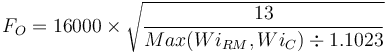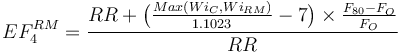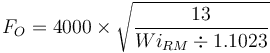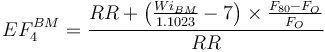# Model:Bond/Barratt SS SAG

## Bond/Barratt Specific Energy Consumption Single-Stage SAG Model

This is a SAG or AG mill plus ball mill model that estimates the SAG mill specific energy consumption (ESAG) using the 1979 Barratt equation.

### Required parameters

• F80, µm is the 80% passing size of the fresh feed to the circuit (expected to be a Bond-compatible size distribution).
• P80, µm is the 80% passing size of the circuit product (expected to be a Bond-compatible size distribution).
• Availability, expressed as a decimal (0.90 = 90% availability) is used to convert t/h to t/d.

### Optional parameters

• Description and Comment are optional text fields
• Maximum t/h limit is a t/h throughput above which a warning message is displayed (but does not actually limit the throughput).
• Ball mill work index adjustment used to adjust WiBM for different P80 sizes.
• coefficient (a), the fitted Hukki coefficient to the adjustment equation (enter as a positive number)
• (calibration) Easag crushing circuit product, µm, model tuning factor for that adjusts the relative weight of the WiC and WiRM
• (calibration) Easag RM circuit product, µm :, model tuning factor for that adjusts the relative weight of the WiRM and WiBM
• CFsag Calibration Factor, is a manual adjustment to the model prediction. This value is multiplied by the prediction, so 1.0 means use the predicted value, 1.5 means use 50% more than the predicted value.

### Formulae\begin{align} E_{SAG} = \Big[ & Wi_{C} \times \left ( \tfrac {10}{\sqrt{ P_{C} }} - \tfrac {10}{\sqrt{ F_{80} }} \right ) + Wi_{RM} \times \left ( \tfrac {10}{\sqrt{ P_{R} }} - \tfrac {10}{\sqrt{ P_{C} }} \right ) \times EF_4^{RM} \\ & + Wi_{BM} \times \left ( \tfrac {10}{\sqrt{110} } - \tfrac {10}{\sqrt{ P_{R} }} \right ) \times EF_4^{BM} \Big] \times 1.25 - Wi_{BM} \times \left ( \tfrac {10}{\sqrt{ {110} }} - \tfrac {10}{\sqrt{ T_{80} }} \right ) \end{align} Barratt, 1989

The optimum feed size for the rod mill oversize feed factor (EF4RM) in the Barratt ESAG equation is calculated using the greater of the sample's rod mill or crushing work index.$F_{O}= 16000 \times \sqrt{\frac{13}{Max(Wi_{RM}, Wi_{C}) \div 1.1023} }$

The EF4RM is calculated greater of the crushing work index or the rod mill work index.$EF_{4}^{RM} = \frac{RR+ \bigl(\frac{Max(Wi_C, Wi_{RM})}{1.1023} -7 \bigr) \times \frac {F_{80}-F_{O}}{F_{O}}}{RR}$

The ball mill oversize feed factor (EF4BM) is always calculated with the rod mill work index for the optimum feed size and the ball mill work index for EF4BM.$F_{O}= 4000 \times \sqrt{\frac{13}{Wi_{RM} \div 1.1023} }$$EF_{4}^{BM} = \frac{RR+ \bigl( \frac{Wi_{BM}}{1.1023} -7 \bigr) \times \frac {F_{80}-F_{O}}{F_{O}}}{RR}$

## Recommended Usage

### Discussion, pebble crushing

Barratt's equation did not consider pebble crushing; the practise was not common when his equation was being calibrated. Any pebble crusher is assumed to be part of the SAG mill for the purposes of this calculation and is assumed to operate at the same efficiency as the SAG mill itself. If you wish to model an improved SAG energy consumption due to pebble crushing, adjust the CFsag Calibration Factor to, for example, 0.95 for an assumed 5% benefit.

### Discussion, Rowland EF factors

The model is only interested in the EF factors that are properties of the rock, namely the coarse feed EF4 factor and the fine product EF5 factor. The EF factors that correspond to machine characteristics are not included (EF1, EF2, EF3, EF6, EF7 and EF8) as they are intended to capture the inefficiency of certain types of milling circuits. The assumption in this model is that the Essbm is the "maximum efficiency" possible for a grinding circuit, to which we apply an empirical adjustment for SAG milling (Cf) which encapsulates any other EF factors in a form suitable for a SAG process.

### Model defaults

• (calibration) Easag crushing circuit product, µm= 9400
• (calibration) Easag RM circuit product, µm= 2100
• CFsag Calibration Factor = 0.10